1. /
2. CBSE
3. /
4. Class 05
5. /
6. Mathematics
7. /
8. NCERT Solutions for Class...

# NCERT Solutions for Class 5 Maths Shapes and Angles### myCBSEguide App

Download the app to get CBSE Sample Papers 2023-24, NCERT Solutions (Revised), Most Important Questions, Previous Year Question Bank, Mock Tests, and Detailed Notes.

NCERT solutions for Class 5 Mathematics## NCERT Solutions for Class 5 Maths Shapes and Angles

###### PRACTICE TIME:

(1) Look at the shape and the answer.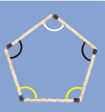The angle marked in ________ colour is the biggest angle.

Ans.  The angle marked in black colour is the biggest angle.

###### 2 (a) Are the angles marked with yellow equal? ________

(b) Are the angles marked with green equal? ________

(c) Are the angles marked with blue equal? ________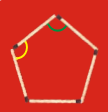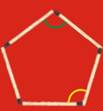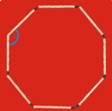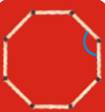Ans. (a) Yes, the angles marked with yellow are equal.

(b) Yes, the angles marked with green are equal.

(c) Yes, the angles marked with blue are equal.

NCERT Solutions for Class 5 Maths Shapes and Angles

###### 3. Four different angles are marked in four colours. Can you find other angles which are the same as the one marked in red? Mark them in red. Do this for the other colours.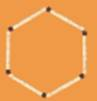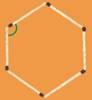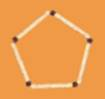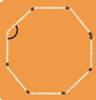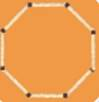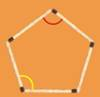Sol. Yes, the marked in yellow is the same as the red one. Other angles which are the same as the one marked in red are marked red in the figure.

###### 4. Sukhman made this picture with so many angles.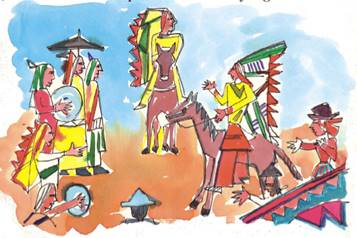Use colour pencils to mark

1. Right angles with black colour.

2. Angles which are more than a right angle with green.

3. Angles which are less than a right angle with blue.

Ans. (1) In the above figures right angles are marked with letter ’R’.

(2) Angles which are more than a right angle are marked with letter ‘M’.

(3) Angles which are less than a right angle marked with letter ‘L’.

NCERT Solutions for Class 5 Maths Shapes and Angles

###### 5.Draw anything of your choice around the angle shown. Also write what kind of angle it is. The first one is done.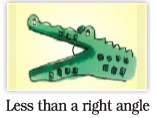Ans.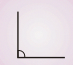A right angle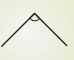A right angle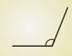More than a right angle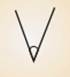Less than a right angle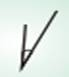Less than a right angle

###### 6.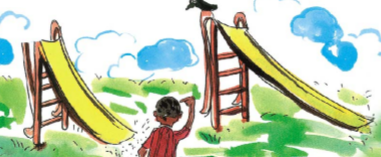These are two slide in a park.

1. Which slide has a larger angle?

2. Which slide do you think is safer for the little boy? Why?

Ans. (1) The slide on the left side has a larger angle.

(2) The slide on the right side is safer for the little boy because of its smaller angle.

NCERT Solutions for Class 5 Maths Shapes and Angles

###### 7. Write what kind of angle is made by the hands at these times. Also write the time.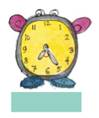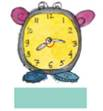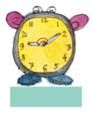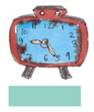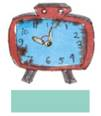Ans. Let these watches be marked as (a), (b), (c), (d)and(e) as shown above. The time given in the following table.

 Clock Angles made by the hands Time (a) Less than a right angle 25 minutes past 7 (b) More than a right angle Quarter past 8 (c) More than a right angle 10 minutes past 9 (d) More than a right angle 25 minutes past 9 (e) Less than a right angle 10’o clock

## NCERT Solutions for Class 5 Mathematics Chapter 2 Shapes and Angles

NCERT Solutions Class 5 Maths PDF (Download) Free from myCBSEguide app and myCBSEguide website. Ncert solution class 5 Maths includes text book solutions from Class 5 Maths Book . NCERT Solutions for CBSE Class 5 Maths have total 14 chapters. 5 Maths NCERT Solutions in PDF for free Download on our website. Ncert Maths class 5 solutions PDF and Maths ncert class 5 PDF solutions with latest modifications and as per the latest CBSE syllabus are only available in myCBSEguide.

## CBSE app for Students

To download NCERT Solutions for Class 5 Maths, Social Science Computer Science, Home Science, Hindi English, Maths Science do check myCBSEguide app or website. myCBSEguide provides sample papers with solution, test papers for chapter-wise practice, NCERT solutions, NCERT Exemplar solutions, quick revision notes for ready reference, CBSE guess papers and CBSE important question papers. Sample Paper all are made available through the best app for CBSE students and myCBSEguide website.Test Generator

Create question paper PDF and online tests with your own name & logo in minutes.myCBSEguide

Question Bank, Mock Tests, Exam Papers, NCERT Solutions, Sample Papers, Notes

### 3 thoughts on “NCERT Solutions for Class 5 Maths Shapes and Angles”

1. Can I write the and

2. Can I write the ans

3. Can I write the ans for class 5